xMolar absorptivityEncyclopedia
The molar absorption coefficient, molar
Mole (unit)
The mole is a unit of measurement used in chemistry to express amounts of a chemical substance, defined as an amount of a substance that contains as many elementary entities as there are atoms in 12 grams of pure carbon-12 , the isotope of carbon with atomic weight 12. This corresponds to a value...

extinction coefficient
, or molar absorptivity, is a measurement of how strongly a chemical species
Chemical species
Chemical species are atoms, molecules, molecular fragments, ions, etc., being subjected to a chemical process or to a measurement. Generally, a chemical species can be defined as an ensemble of chemically identical molecular entities that can explore the same set of molecular energy levels on a...

absorbs light at a given wavelength
Wavelength
In physics, the wavelength of a sinusoidal wave is the spatial period of the wave—the distance over which the wave's shape repeats.It is usually determined by considering the distance between consecutive corresponding points of the same phase, such as crests, troughs, or zero crossings, and is a...

. It is an intrinsic property of the species; the actual absorbance, A, of a sample is dependent on the pathlength, , and the concentration, c, of the species via the Beer–Lambert law,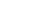.

The SI units
Si
Si, si, or SI may refer to :- Measurement, mathematics and science :* International System of Units , the modern international standard version of the metric system...

for ε are m2/mol, but in practice, they are usually taken as M−1 cm−1 or L mol−1 cm−1. In older literature, cm2 mol−1 is sometimes used with corresponding values 1000 times larger. These units may look different, but it is just a matter of expressing volume in cm3 or in L.

Different disciplines have different conventions as to whether absorbance is Naperian or decadic, i.e., defined with respect to the transmission via natural or common logarithm. The molar absorption coefficient is usually decadic, but when ambiguity exists it is best to qualify it as such.

The molar extinction coefficient should not be confused with the different definition of "extinction coefficient" used more commonly in physics, namely the imaginary part of the complex index of refraction (which is unitless). In fact, they have a straightforward but nontrivial relationship; see Mathematical descriptions of opacity
Mathematical descriptions of opacity
When an electromagnetic wave travels through a medium in which it gets absorbed , it undergoes exponential decay as described by the Beer–Lambert law. However, there are many possible ways to characterize the wave and how quickly it is absorbed...

.

In biochemistry
Biochemistry
Biochemistry, sometimes called biological chemistry, is the study of chemical processes in living organisms, including, but not limited to, living matter. Biochemistry governs all living organisms and living processes...

, the extinction coefficient of a protein
Protein
Proteins are biochemical compounds consisting of one or more polypeptides typically folded into a globular or fibrous form, facilitating a biological function. A polypeptide is a single linear polymer chain of amino acids bonded together by peptide bonds between the carboxyl and amino groups of...

at 280 nm depends almost exclusively on the number of aromatic residues, particularly tryptophan
Tryptophan
Tryptophan is one of the 20 standard amino acids, as well as an essential amino acid in the human diet. It is encoded in the standard genetic code as the codon UGG...

, and can be predicted from the sequence of amino acids. If the extinction coefficient is known, it can be used to determine the concentration of a protein in solution.

When there is more than one absorbing species in a solution, the overall absorbance is the sum of the absorbances for each individual species (X, Y, etc.):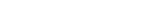,

The composition of a mixture of N components can be found by measuring the absorbance at N wavelength
Wavelength
In physics, the wavelength of a sinusoidal wave is the spatial period of the wave—the distance over which the wave's shape repeats.It is usually determined by considering the distance between consecutive corresponding points of the same phase, such as crests, troughs, or zero crossings, and is a...

s (the values of ε for each compound at these wavelengths must also be known). The wavelengths chosen are usually the wavelengths of maximum absorption (absorbance maxima) for the individual components. None of the wavelengths must be an isosbestic point
Isosbestic point
In spectroscopy, an isosbestic point is a specific wavelength at which two chemical species have the same molar absorptivity or -more generally- are linearly related...

for a pair of species. For N components with concentrations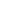and wavelengths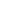, absorbances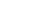are obtained: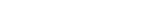.

This set of simultaneous equations
Simultaneous equations
In mathematics, simultaneous equations are a set of equations containing multiple variables. This set is often referred to as a system of equations. A solution to a system of equations is a particular specification of the values of all variables that simultaneously satisfies all of the equations...

can be solved to find concentrations of each absorbing species.

The molar extinction coefficient(if expressed in units of L mol−1 cm−1) is directly related to the Absorption cross section
Absorption cross section
Absorption cross section is a measure for the probability of an absorption process. More generally, the term cross section is used in physics to quantify the probability of a certain particle-particle interaction, e.g., scattering, electromagnetic absorption, etc...

,, (in units of cm2) via the Avogadro constant: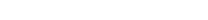.

The molar absorptivity is also closely related to the mass attenuation coefficient
Mass attenuation coefficient
The mass attenuation coefficient is a measurement of how strongly a chemical species or substance absorbs or scatters light at a given wavelength, per unit mass...

, by the equation×(Molar mass
Molar mass
Molar mass, symbol M, is a physical property of a given substance , namely its mass per amount of substance. The base SI unit for mass is the kilogram and that for amount of substance is the mole. Thus, the derived unit for molar mass is kg/mol...

) = (Molar absorptivity).

## See also

• Molar extinction coefficient of dansyl compounds Dansyl chloride
Dansyl chloride
Dansyl chloride or 5-naphthalene-1-sulfonyl chloride is a reagent that reacts with primary amino groups in both aliphatic and aromatic amines to produce stable blue- or blue-green–fluorescent sulfonamide adducts. Dansyl chloride is widely used to modify amino acids; specifically, protein sequencing...

• Quantum yield
Quantum yield
The quantum yield of a radiation-induced process is the number of times that a defined event occurs per photon absorbed by the system. The "event" may represent a chemical reaction, for example the decomposition of a reactant molecule:...

; the product of quantum yield and molar absorptivity gives a brightness for fluorescent dyes.

## External links

The source of this article is wikipedia, the free encyclopedia.  The text of this article is licensed under the GFDL.